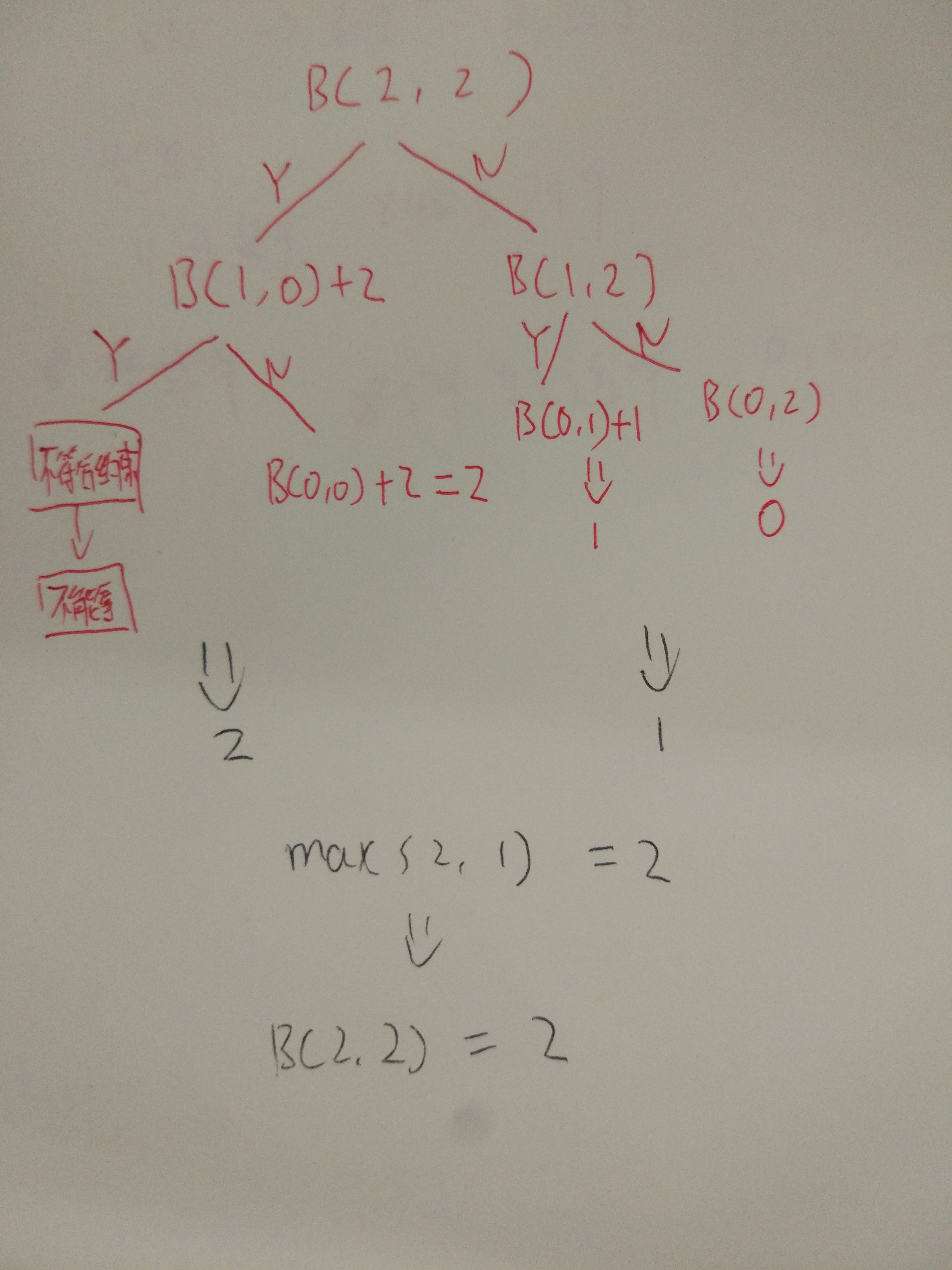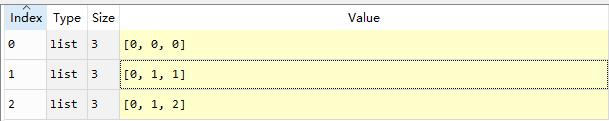# 一文彻底搞懂01背包算法

0-1 背包问题：给定 n 种物品和一个容量为 C 的背包，物品 i 的重量是 ${w}_{i}$$w_i$，其价值为 ${v}_{i}$$v_i$

$max.F\left(n,C,x\right).x\in 0,1$

$F\left(n,C,x\right)={x}_{1}\ast {v}_{1}+{x}_{2}\ast {v}_{2}+....+{x}_{n}\ast {v}_{n}$
$std.{x}_{1}\ast {w}_{1}+x2\ast w2+...+{x}_{n}\ast {w}_{n}\le C$
${x}_{i}\in 0,1$

x的取值范围为0或者1，代表着这个物品我们可以选择拿或者不拿，我们想要找出这样的01组合如：$（1，1，1，0，0，1）$$（1，1，1，0，0，1）$使得$F\left(n,C,x\right)$$F(n,C,x)$最大。

$B\left(n,C\right)=max.F\left(n,c,x\right)$

$B\left(n,c\right)=B\left(n-1,c\right);没有多余的空间去放置最后一个物品$
$B\left(n,c\right)=max\left\{B\left(n-1,c\right),B\left(n-1,c-{w}_{n}\right)+{v}_{n}\right\};如果有多余的空间去放置，则考虑是否要放置$

1、拿：如果确定拿走最后一个物品，则B(2,2)=B(2-1,2-2)+2=B(1,0)+2
2、不拿：如果确定不拿走最后一个物品，则B(2,2)=B(1,2);因为最后一个物品我选择不拿，所以情景肯定变为从1个物品里面选，容量为2，是否达到最大值，因此等式左右两边相等。

$B\left(2,2\right)=max\left\{B\left(1,0\right)+2,B\left(1,0\right)+2\right\}=max\left\{2,1\right\}=2$$B(2,2)=max\{B(1,0)+2,B(1,0)+2\}=max\{2,1\}=2$capicaty=[0,1,2]
value=[0,1,2]

def  best_value(i,j):
if i==0:
return 0
if j-capicaty[i]<0:
return best_value(i-1,j)
else:
return max(best_value(i-1,j),best_value(i-1,j-capicaty[i])+value[i])

print(best_value(2,2))

result:2  

maxvalue=[[0 for i in range(3)] for j in range(3)]
for k in range(1,3):
for c in range(1,3):
if c-capicaty[k]>=0:
maxvalue[k][c]=max(maxvalue[k-1][c],maxvalue[k-1][c-capicaty[k]]+value[k])
else:
maxvalue[k][c]=maxvalue[k-1][c]B(2,2)那么我们去索引，maxvalue同样得到2的结果,这样的话这个算法你就掌握了，可以去到动态规划的世界玩耍一番，好好感悟一下，晦涩难懂的理论知识，状态，状态转移方程，把目标划分为子目标，分而治之，你会发现你看得懂了，完美。